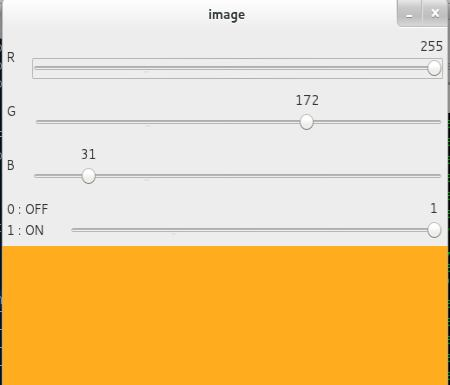# 作为调色板的跟踪栏

## 代码演示

import numpy as np
import cv2 as cv
def nothing(x):
pass
# 创建一个黑色图像，一个窗口
img = np.zeros((300,512,3), np.uint8)
cv.namedWindow('image')
# 创建一个改变颜色的轨迹栏
cv.createTrackbar('R','image',0,255,nothing)
cv.createTrackbar('G','image',0,255,nothing)
cv.createTrackbar('B','image',0,255,nothing)
# 创建一个用来启用和关闭功能的开关
switch = '0 : OFF \n1 : ON'
cv.createTrackbar(switch, 'image',0,1,nothing)
while(1):
cv.imshow('image',img)
k = cv.waitKey(1) & 0xFF
if k == 27:
break
# get current positions of four trackbars
r = cv.getTrackbarPos('R','image')
g = cv.getTrackbarPos('G','image')
b = cv.getTrackbarPos('B','image')
s = cv.getTrackbarPos(switch,'image')
if s == 0:
img[:] = 0
else:
img[:] = [b,g,r]
cv.destroyAllWindows()## 练习

1. 创建一个 Paint 程序，具有可调节颜色和笔刷半径的轨迹栏。关于绘画，参考之前的鼠标事件教程。